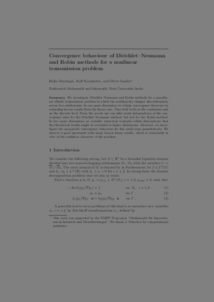Repository: Freie Universität Berlin, Math Department

# Convergence behaviour of Dirichlet-Neumann and Robin methods for a nonlinear transmission problem

Berninger, H. and Kornhuber, R. and Sander, O. (2011) Convergence behaviour of Dirichlet-Neumann and Robin methods for a nonlinear transmission problem. In: Domain Decomposition Methods in Science and Engineering XIX. Lecture Notes in Computational Science and Engineering, 78 . Springer Berlin Heidelberg, pp. 87-98. ISBN 978-3-642-11303-1Preview

2MB

Official URL: http://dx.doi.org/10.1007/978-3-642-11304-8_8

## Abstract

We investigate Dirichlet–Neumann and Robin methods for a quasilinear elliptic transmission problem in which the nonlinearity changes discontinuously across two subdomains. In one space dimension, we obtain convergence theorems by extending known results from the linear case. They hold both on the continuous and on the discrete level. From the proofs one can infer mesh-independence of the convergence rates for the Dirichlet–Neumann method, but not for the Robin method. In two space dimensions, we consider numerical examples which demonstrate that the theoretical results might be extended to higher dimensions. Moreover, we investigate the asymptotic convergence behaviour for fine mesh sizes quantitatively. We observe a good agreement with many known linear results, which is remarkable in view of the nonlinear character of the problem.

Item Type: Book Section Mathematical and Computer Sciences > Mathematics > Numerical Analysis Department of Mathematics and Computer Science > Institute of Mathematics 1797 Ekaterina Engel 18 Feb 2016 09:37 03 Mar 2017 14:41

Repository Staff Only: item control page